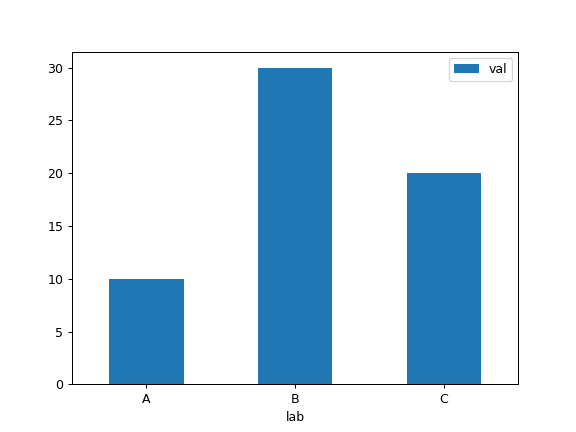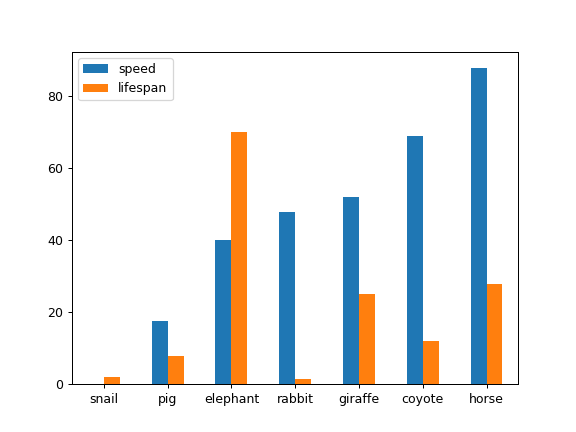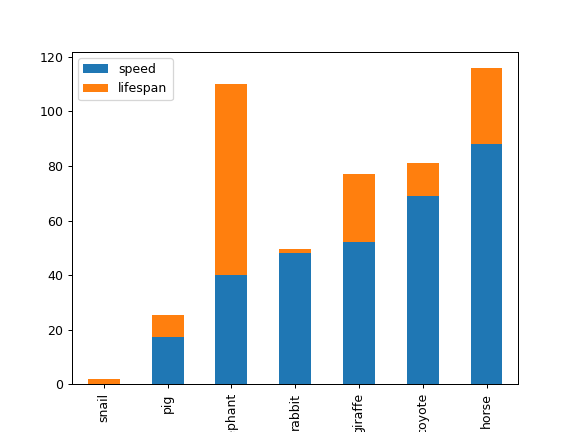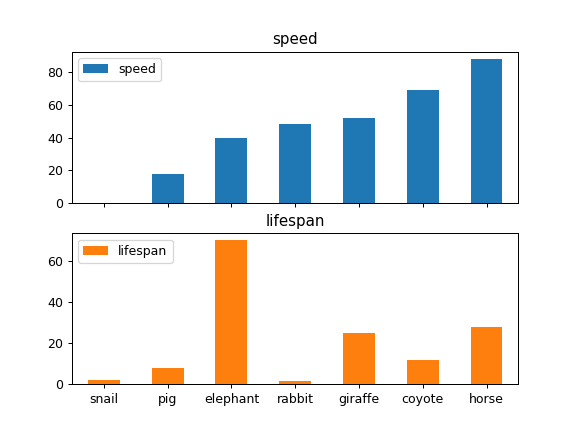# pandas.DataFrame.plot.bar¶

DataFrame.plot.bar(self, x=None, y=None, **kwargs)[source]

Vertical bar plot.

A bar plot is a plot that presents categorical data with rectangular bars with lengths proportional to the values that they represent. A bar plot shows comparisons among discrete categories. One axis of the plot shows the specific categories being compared, and the other axis represents a measured value.

Parameters: x : label or position, optional Allows plotting of one column versus another. If not specified, the index of the DataFrame is used. y : label or position, optional Allows plotting of one column versus another. If not specified, all numerical columns are used. **kwds Additional keyword arguments are documented in DataFrame.plot(). matplotlib.axes.Axes or np.ndarray of them An ndarray is returned with one matplotlib.axes.Axes per column when subplots=True.

DataFrame.plot.barh
Horizontal bar plot.
DataFrame.plot
Make plots of a DataFrame.
matplotlib.pyplot.bar
Make a bar plot with matplotlib.

Examples

Basic plot.

>>> df = pd.DataFrame({'lab':['A', 'B', 'C'], 'val':[10, 30, 20]})
>>> ax = df.plot.bar(x='lab', y='val', rot=0)Plot a whole dataframe to a bar plot. Each column is assigned a distinct color, and each row is nested in a group along the horizontal axis.

>>> speed = [0.1, 17.5, 40, 48, 52, 69, 88]
>>> lifespan = [2, 8, 70, 1.5, 25, 12, 28]
>>> index = ['snail', 'pig', 'elephant',
...          'rabbit', 'giraffe', 'coyote', 'horse']
>>> df = pd.DataFrame({'speed': speed,
...                    'lifespan': lifespan}, index=index)
>>> ax = df.plot.bar(rot=0)Instead of nesting, the figure can be split by column with subplots=True. In this case, a numpy.ndarray of matplotlib.axes.Axes are returned.

>>> axes = df.plot.bar(rot=0, subplots=True)
>>> axes.legend(loc=2)  # doctest: +SKIPPlot a single column.

>>> ax = df.plot.bar(y='speed', rot=0)Plot only selected categories for the DataFrame.

>>> ax = df.plot.bar(x='lifespan', rot=0)Scroll To Top